Question

# For each of the following notes receivable, compute the amount of interest revenue eamed during 2018. Use a 360-da...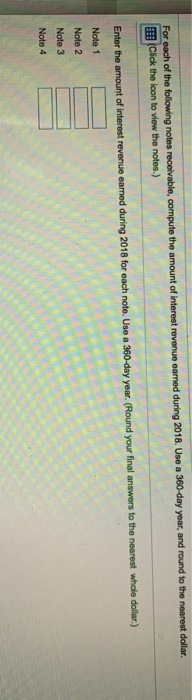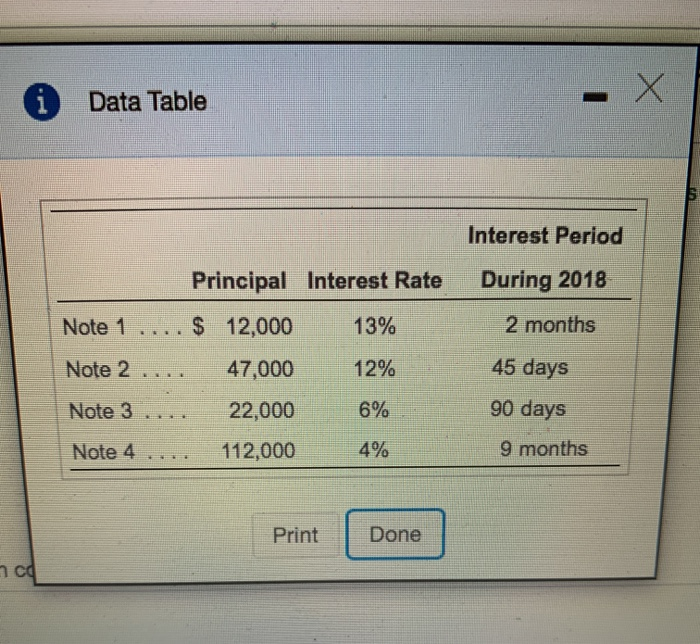For each of the following notes receivable, compute the amount of interest revenue eamed during 2018. Use a 360-day year, and round to the nearest dollar Click the icon to view the notes.) Enter the amount of interest revenue earned during 2018 for each note. Use a 360-day year. (Round your final answers to the nearest whole dollar.) Note 1 Note 2 Note 3 Note 4
i Data Table Interest Period During 2018 2 months Principal Interest Rate Note 1 .... \$ 12,000 13% Note 2 .... 47,000 12% Note 3 22,000 6% Note 4 112,000 45 days 90 days 4% 9 months Print Done 7cd

Interest revenue earned on Note 1 = 12,000 x 13% x 2/12

= \$260

Interest revenue earned on Note 2 = 47,000 x 12% x 45/360

= \$705

Interest revenue earned on Note 3 = 22,000 x 6% x 90/360

= \$330

Interest revenue earned on Note 4 = 112,000 x 4% x 9/12

= \$3,360

 Note 1 \$260 Note 2 \$705 Note 3 \$330 Note 4 \$3,360

Kindly give a positive rating if you are satisfied with this solution and please ask if you have any query.

Thanks

#### Earn Coins

Coins can be redeemed for fabulous gifts.

Similar Homework Help Questions
• ### For each of the following notes receivable, compute the amount of interest revenue earned during 2018....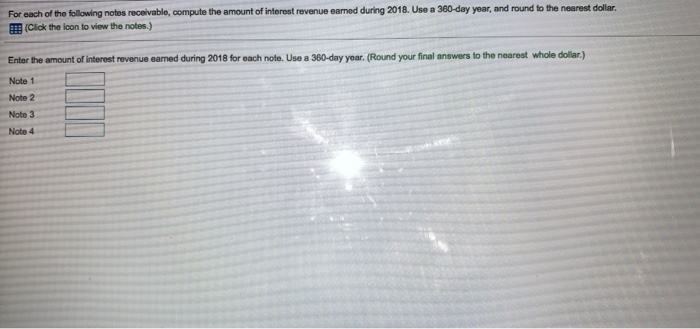For each of the following notes receivable, compute the amount of interest revenue earned during 2018. Use a 360-day year, and round to the nearest dollar. (Click the icon to view the notes.) Enter the amount of interest revenue eamed during 2018 for each note. Use a 360-day year. (Round your final answers to the nearest whole dollar) Note 1 Note 2 Note 3 Note 4 rest revenue eamed during 2018. Use a 360-day year, and round to the nearest...

• ### For each of the following notes receivable, compute the amount of (Click the icon to view...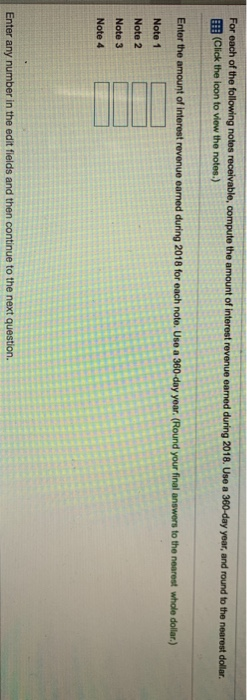For each of the following notes receivable, compute the amount of (Click the icon to view the notes.) revenue eamed during 2018. Use a 360-day year, and round to the nearest dollar. Enter the amount of interest revenue earned during 2018 for each note. Use a 360-day year. (Round your final answers to the nearest whole dollar.) Note 1 Note 2 Note 3 Note 4 Enter any number in the edit fields and then continue to the next question Compu...

• ### For each of the following notes receivable, compute the amount of interest revenue earned during 2020....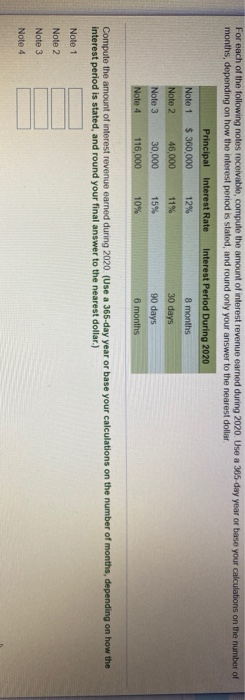For each of the following notes receivable, compute the amount of interest revenue earned during 2020. Use a 365 day year or base your calculations on the number of months, depending on how the interest period is stated, and round only your answer to the nearest dollar Note 1 Note 2 Note 3 Note 4 Principal interest Rate \$360,000 12% 46,000 30,000 15% 116,000 10% Interest Period During 2020 8 months 30 days 90 days 6 months Compute the amount...

• ### A table of notes receivable for 2018 follows: : (Click the icon to view the table...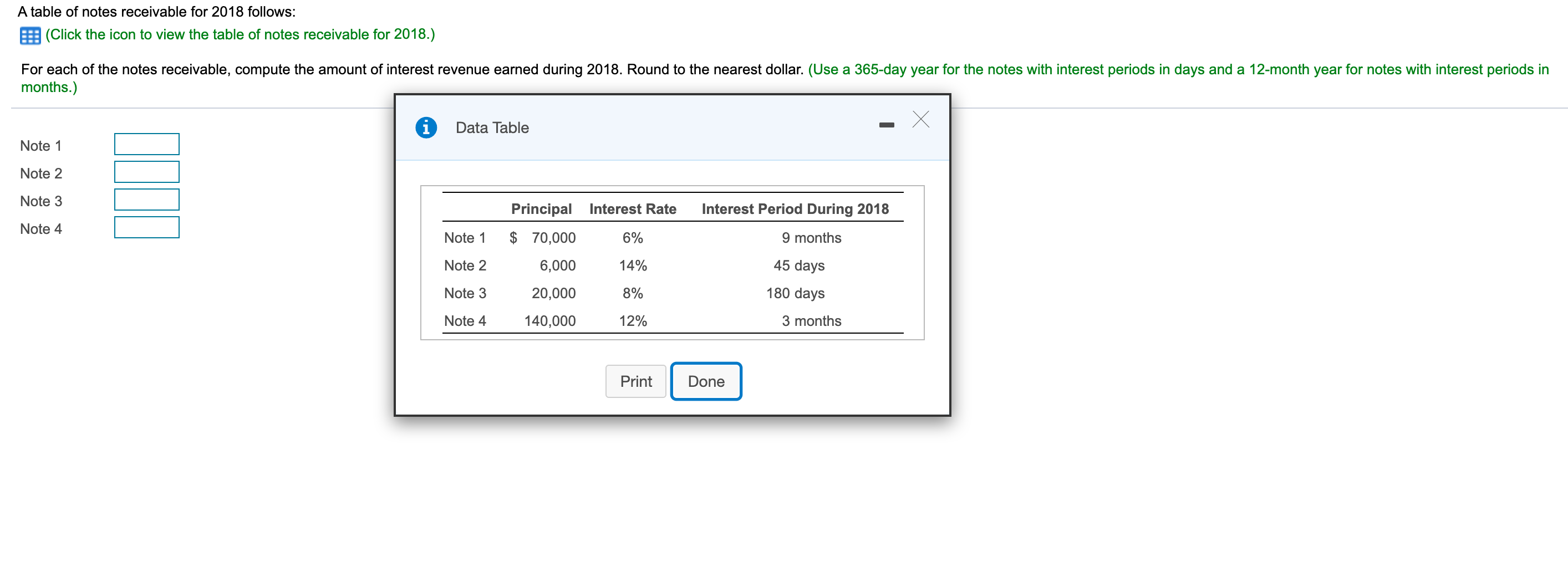A table of notes receivable for 2018 follows: : (Click the icon to view the table of notes receivable for 2018.) For each of the notes receivable, compute the amount of interest revenue earned during 2018. Round to the nearest dollar. (Use a 365-day year for the notes with interest periods in days and a 12-month year for notes with interest periods in months.) Data Table Note 1 Note 2 Note 3 Interest Period During 2018 Note 4 9 months...

• ### Computing Accrued Interest Compute the interest accrued on each of the following notes receivable held by...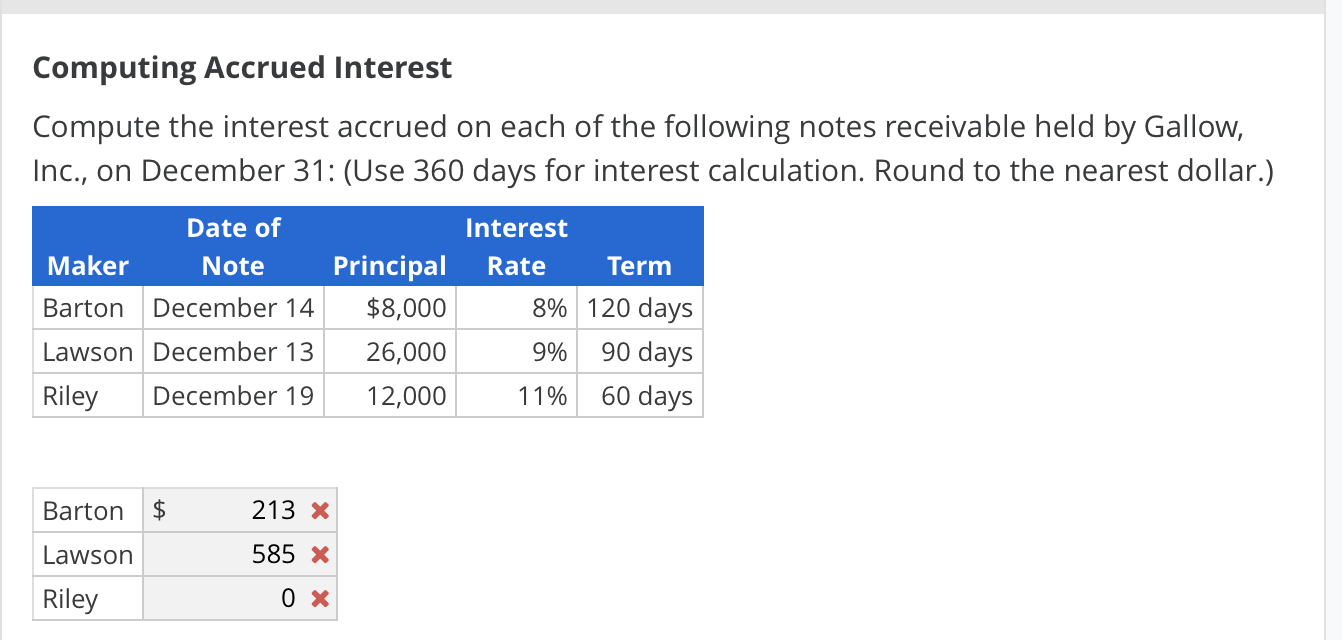Computing Accrued Interest Compute the interest accrued on each of the following notes receivable held by Gallow, Inc., on December 31: (Use 360 days for interest calculation. Round to the nearest dollar.) Date of Maker Note Barton December 14 Lawson December 13 Riley December 19 Interest Principal Rate Term \$8,000 8% 120 days 26,000 9% 90 days 12,000 11% 60 days \$ Barton Lawson 213 X 585 x 0 x Riley

• ### Maturity Dates of Notes Receivable Determine the maturity date and compute the interest for each of...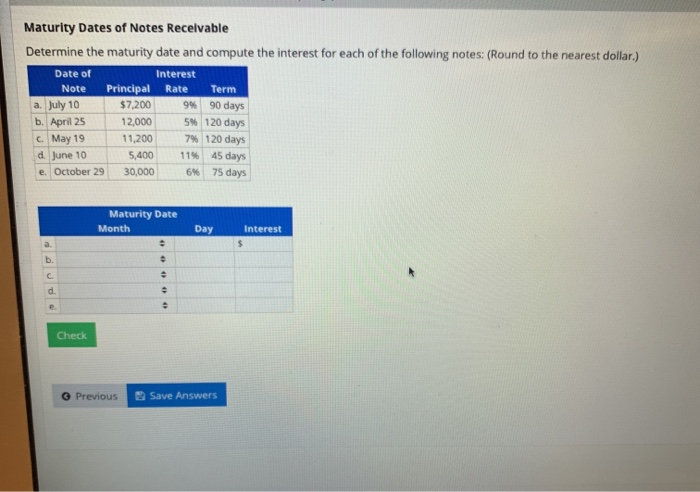Maturity Dates of Notes Receivable Determine the maturity date and compute the interest for each of the following notes: (Round to the nearest dollar.) Date of Interest Note Principal Rate Term a. July 10 \$7,200 94 90 days b. April 25 12,000 5 120 days May 19 11,200 7% 120 days d June 10 5.400 1145 days e. October 29 30,000 6% 75 days Maturity Date Month Day Interest Check Previous Save Answers

• ### Maturity Dates of Notes Receivable Determine the maturity date and compute the interest for each of...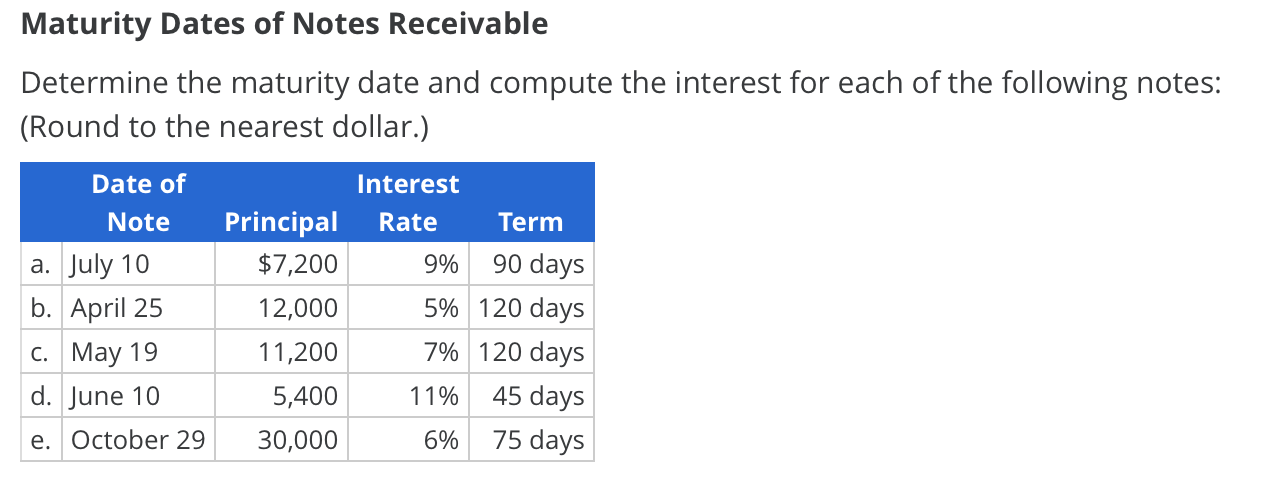Maturity Dates of Notes Receivable Determine the maturity date and compute the interest for each of the following notes: (Round to the nearest dollar.) Date of Interest Principal Rate Note Term 90 days \$7,200 а. July 10 9% 5% 120 days b. April 25 12,000 7% 120 days C. May 19 11,200 45 days d. June 10 5,400 11% 75 days e. October 29 30,000 6%

• ### Exercise 9-4 Interest-bearing notes payable with year-end adjustments LO P

Exercise 9-4 Interest-bearing notes payable with year-end adjustments LO P Keesha Co. borrows \$160,000 cash on November 1, 2017, by signing a 120-day, 8% note with a face value of \$160,000. 1. On what date does this note mature? (Assume that February has 28 days) March 27, 2018. March 28, 2018. March 29, 2018. March 30, 2018. *March 01, 2018 2.& 3. What is the amount of Interest expense in 2017 and 2018 from this note? (Use 360 days a year. Round-final answers to the nearest whole...

• ### Moses Supply Co. has the following transactions related to notes receivable during the last 2 months...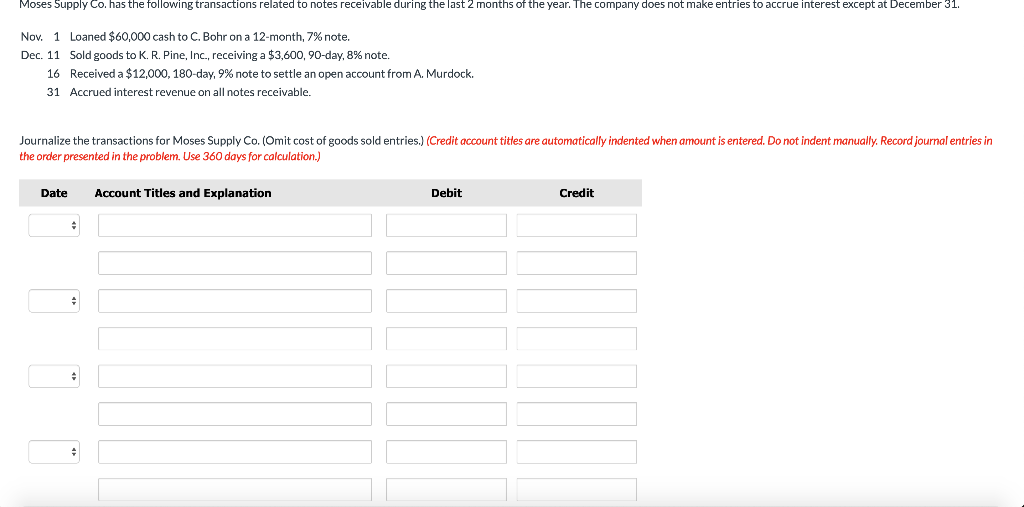Moses Supply Co. has the following transactions related to notes receivable during the last 2 months of the year. The company does not make entries to accrue interest except at December 31. Nov. 1 Loaned \$60,000 cash to C. Bohr on a 12-month, 7% note. Dec. 11 Sold goods to K. R. Pine, Inc., receiving a \$3,600,90-day, 8% note. 16 Received a \$12,000, 180-day, 9% note to settle an open account from A. Murdock. 31 Accrued interest revenue on all...

• ### Compute interest and find the maturity date for the following notes. (Use 360 days for calculation.)...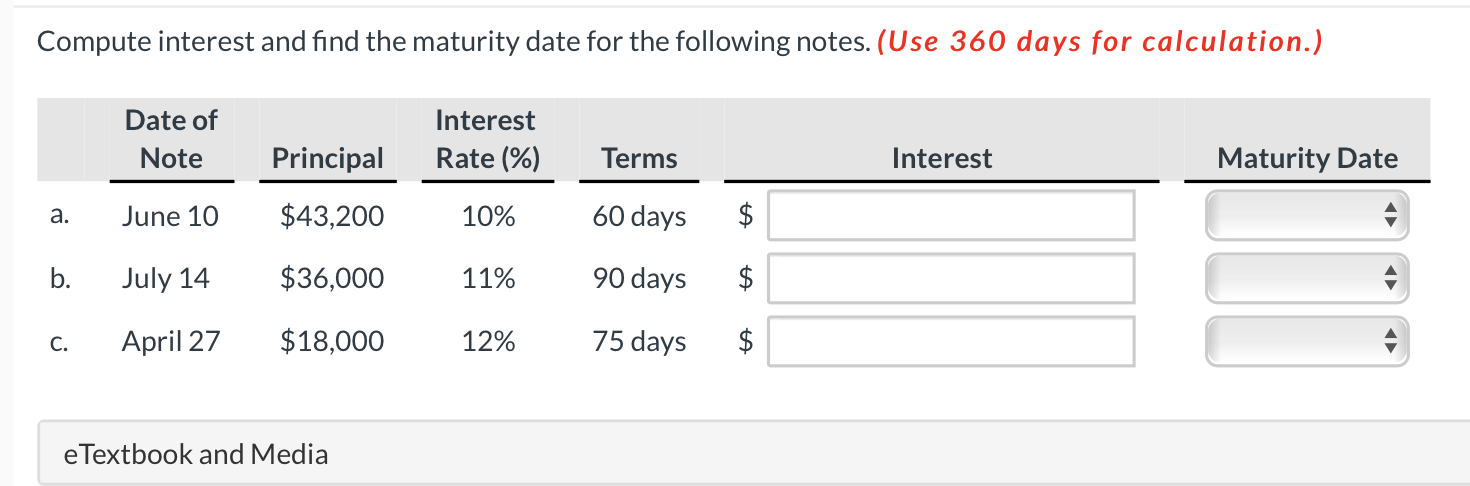Compute interest and find the maturity date for the following notes. (Use 360 days for calculation.) Date of Note Interest Rate (%) Principal Terms Interest Maturity Date a. June 10 \$43,200 10% 60 days \$ b. July 14 \$36,000 11% 90 days \$ C. April 27 \$18,000 12% 75 days \$ \$ e Textbook and Media